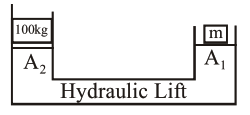# A hydraulic press can`
Question:

A hydraulic press can lift $100 \mathrm{~kg}$ when a mass 'm' is placed on the smaller piston. It can lift $\mathrm{kg}$ when the diameter of the larger piston is increased by 4 times and that of the smaller piston is decreased by 4 times keeping the same mass ' $m$ ' on the smaller piston.

Solution:

Using Pascals law$\frac{100 \times \mathrm{g}}{\mathrm{A}_{2}}=\frac{\mathrm{mg}}{\mathrm{A}_{1}}$...(1)

Let $m$ mass can lift $\mathrm{M}_{0}$ in second case then

$\frac{\mathrm{M}_{0} \mathrm{~g}}{16 \mathrm{~A}_{2}}=\frac{\mathrm{mg}}{\mathrm{A}_{1} / 16}$...(2)

$\left\{\right.$ Since $\left.A=\frac{\pi \mathrm{d}^{2}}{4}\right\}$

From equation (1) and (2) we get

$\frac{\mathrm{M}_{0}}{16.100}=16$

$\Rightarrow \mathrm{M}_{0}=25600 \mathrm{~kg}$